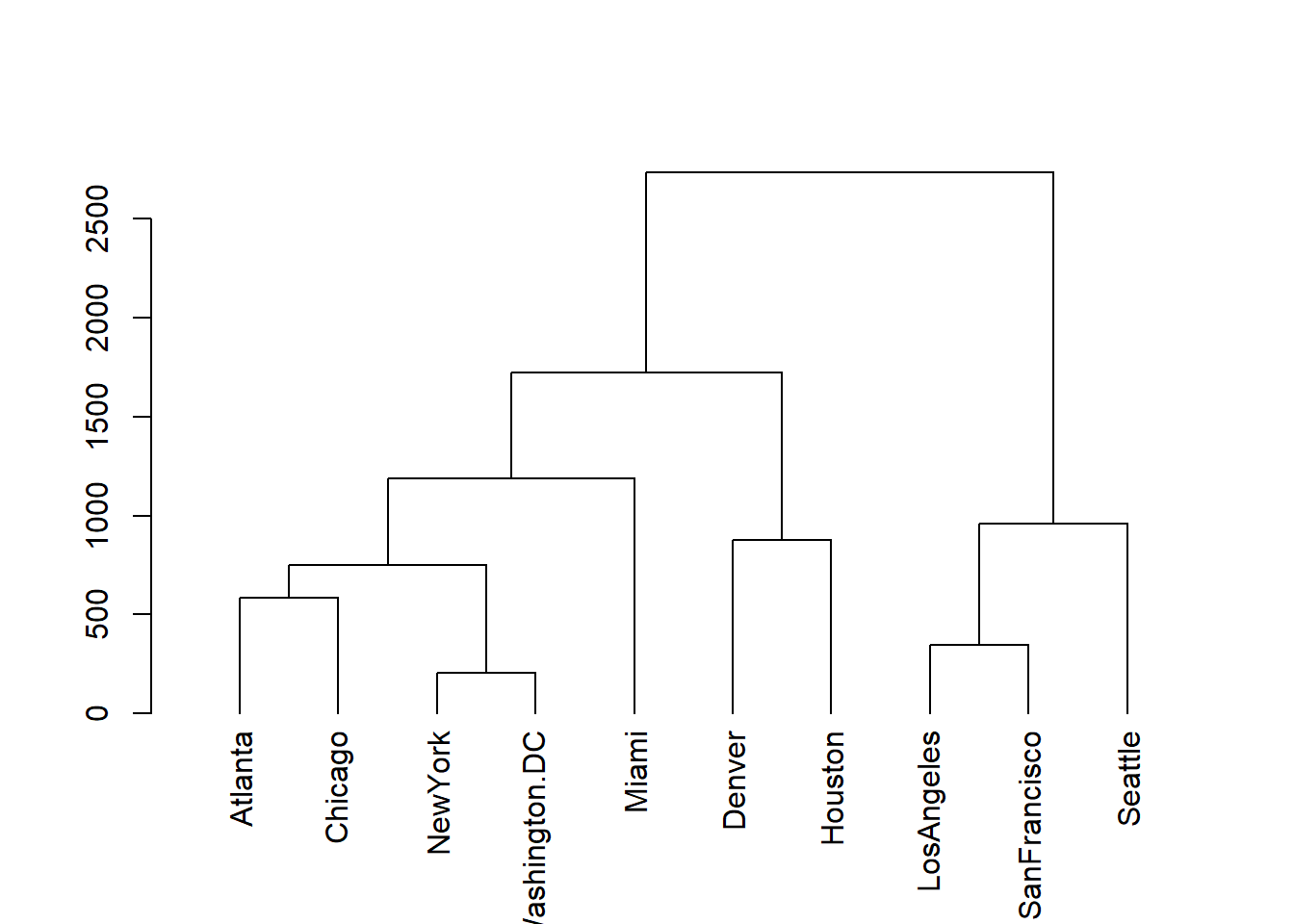Description

R package mdendro enables the calculation of agglomerative hierarchical clustering (AHC), extending the standard functionalities in several ways:

• Native handling of both similarity and dissimilarity (distances) matrices.

• Calculation of pair-group dendrograms and variable-group multidendrograms .

• Implementation of the most common AHC methods in both weighted and unweighted forms: single linkage, complete linkage, average linkage (UPGMA and WPGMA), centroid (UPGMC and WPGMC), and Ward.

• Calculation of the cophenetic (or ultrametric) matrix.

• Calculation of five descriptors of the final dendrogram: cophenetic correlation coefficient, space distortion ratio, agglomerative coefficient, chaining coefficient, and tree balance.

• Plots of the descriptors for the parametric methods.

All this functionality is obtained with two functions: linkage, and descplot. Function linkage may be considered as a replacement for functions hclust (in package stats) and agnes (in package cluster). To enhance usability and interoperability, the linkage class includes several methods for plotting, summarizing information, and class conversion.

Installation

There exist two main ways to install mdendro:

• Installation from CRAN (recommended method):

install.packages("mdendro")

RStudio has a menu entry (Tools $$\rightarrow$$ Install Packages) for this job.

• Installation from GitHub (you may need to install first devtools):

install.packages("devtools")
library(devtools)
install_github("sergio-gomez/mdendro")

Since mdendro includes C++ code, you may need to install first Rtools in Windows, or Xcode in MacOS.

Tutorial

Basics

Let us start by using the linkage function to calculate the complete linkage AHC of the UScitiesD dataset, a matrix of distances between a few US cities:

library(mdendro)
lnk <- linkage(UScitiesD, method = "complete")

Now we can plot the resulting dendrogram:

plot(lnk)The summary of this dendrogram is:

summary(lnk)
## Call:
##         type.prox = "distance",
##         digits = 0,
##         method = "complete",
##         group = "variable")
##
## Binary dendrogram: TRUE
##
## Descriptive measures:
##       cor       sdr        ac        cc        tb
## 0.8077859 1.0000000 0.7738478 0.3055556 0.9316262

In particular, you can recognize the calculated descriptors:

• cor: cophenetic correlation coefficient
• sdr: space distortion ratio
• ac: agglomerative coefficient
• cc: chaining coefficient
• tb: tree balance

It is possible to work with similarity data without having to convert them to distances, provided they are in range [0.0, 1.0]. A typical example would be a matrix of non-negative correlations:

sim <- as.dist(Harman23.cor\$cov)
lnk <- linkage(sim, type.prox = "sim")
plot(lnk, main = "Harman23")